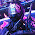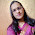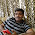## Monday, 29 September 2014

1.It's helping!

2.3.Thank you thank you thank you thank you thank you.........soooooooooo much

4.Thank you 😊

5.You could have just used 1st equation instead of 2 equations in Q7

6.Helpful but ans. Of q.8 a part is wrong as it is not a straight line . So plz see it and put coorect ans.

7.There is no 10th question's solution. Please check it out

8.There is no 10th question's solution. Please check it out

9.There is no 10th question's solution. Please check it out

10.10 question's solution is not seen but the other answers are good and valuable

11.10. Radius = 42250 km.
Distance covered in 1 Revolution = 2× 3.14 × 42250
Time = 24 ×60 ×60
Speed = Distance/ time = 2×3.14×42250 / (24 × 60 ×60) = 3.07 km/s

12.thank you so much

13.stupid network

14.15.In 6th question where for 18 in that question 18 kaha sa aya